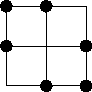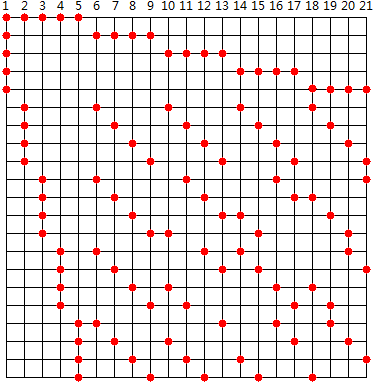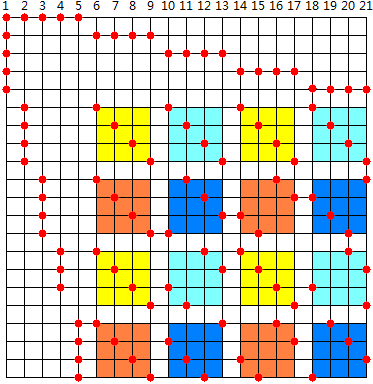# 程序控

IPPP (Institute of Penniless Peasent-Programmer) Fellow

:: :: :: :: :: :: :: ::77 随笔 :: 0 文章 :: 457 评论 :: 0 引用

## Problem问题

Consider a grid such as the one shown. We wish to mark k intersections in each of n rows and n columns in such a way that no 4 of the selected intersections form a rectangle with sides parallel to the grid. Thus for k = 2 and n = 3, a possible solution is:It can easily be shown that for any given value of k, k2-k+1 is a lower bound on the value of n, and it can be shown further that n need never be larger than this.

Write a program that will find a solution to this problem for k32, k-1 will be 0, 1 or prime.

## Input and Output输入与输出

Input will consist of some values for k, one of each line. For each value of k, output will consist of n lines of k points indicating the selected points on that line. Print a blank line between two values of k.

2
1

1 2
1 3
2 3

1

## Analysis分析(i, j, s)为一个三元组，现在要求的就是(0, 0, 0)到(3, 3, 3)，一共4×4×4=64个值。i=0时，规律很明显，(0, j, s)=5 + s * (k - 1) + 1。式中第一个5是空出前面的5个格，s * (k - 1)计算当前4×4方块的起点，后面加1是为了保证数字从1开始，而不是0。

• (j + (s * i)) % m + s * m + k + 1

## Solution解答

#include <iostream>
using namespace std;
//主函数
int main(void) {
//循环处理每一组输入
for (int k, nFirst = 1; cin >> k; nFirst = 0) {
//不是第一次输出时，前面要加空行
if (nFirst == 0) {
cout << endl;
}
int m = k - 1;
//输出头部的k行
for (int i = 0; i < k; ++i) {
cout << 1;
for (int j = 1; j < k; ++j) {
cout << ' ' << i * m + j + 1;
}
cout << endl;
}
//以下算法请参照图示及相关文档
for (int i = 0; i < m; ++i) {
for (int j = 0; j < m; ++j) {
cout << i + 2;
for (int s = 0; s < m; ++s) {
cout << ' ' << (j + (s * i)) % m + s * m + k + 1;
}
cout << endl;
}
}
}
return 0;
}

posted on 2010-08-20 23:40  Devymex  阅读(1347)  评论(0编辑  收藏